Next: Exercises Up: Fundamental Concepts Previous: Uncertainty Relation

# Continuous Spectra

Up to now, we have studiously avoided dealing with observables possessing eigenvalues that lie in a continuous range, rather than having discrete values. The reason for this is that continuous eigenvalues imply a ket space of nondenumerably infinite dimension. Unfortunately, continuous eigenvalues are unavoidable in quantum mechanics. In fact, the most important observables of all--namely position and momentum--generally have continuous eigenvalues. Fortunately, many of the results that we obtained previously for a finite-dimensional ket space with discrete eigenvalues can be generalized to ket spaces of nondenumerably infinite dimensions.

Suppose that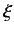is an observable with continuous eigenvalues. We can still write the eigenvalue equation as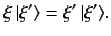(84)

But,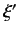can now take a continuous range of values. Let us assume, for the sake of simplicity, thatcan take any value. The orthogonality condition (50) generalizes to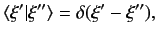(85)

where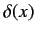denotes the famous Dirac delta function, and satisfies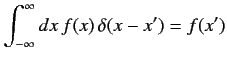(86)

for any function,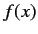, that is well-behaved at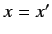. Note that there are clearly a nondenumerably infinite number of mutually orthogonal eigenstates of. Hence, the dimensionality of ket space is nondenumerably infinite. Furthermore, eigenstates corresponding to a continuous range of eigenvalues cannot be normalized such that they have unit norms. In fact, these eigenstates have infinite norms: i.e., they are infinitely long. This is the major difference between eigenstates in a finite-dimensional and an infinite-dimensional ket space. The extremely useful relation (54) generalizes to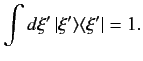(87)

Note that a summation over discrete eigenvalues goes over into an integral over a continuous range of eigenvalues. The eigenstatesmust form a complete set ifis to be an observable. It follows that any general ket can be expanded in terms of the. In fact, the expansions (51)-(53) generalize to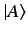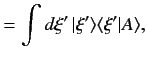(88)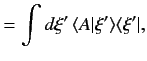(89)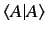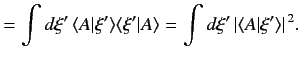(90)

These results also follow simply from Equation (87). We have seen that it is not possible to normalize the eigenstatessuch that they have unit norms. Fortunately, this convenient normalization is still possible for a general state vector. In fact, according to Equation (90), the normalization condition can be written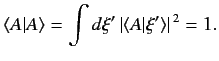(91)

We have now studied observables whose eigenvalues take a discrete number of values, as well as those whose eigenvalues take a continuous range of values. There are a number of other cases we could look at. For instance, observables whose eigenvalues can take a (finite) continuous range of values, plus a set of discrete values. Such cases can be dealt with using a fairly straightforward generalization of the previous analysis (see Dirac, Chapters II and III).Next: Exercises Up: Fundamental Concepts Previous: Uncertainty Relation
Richard Fitzpatrick 2013-04-08